Get inspired by the success stories of our students in IIT JAM MS, ISI  MStat, CMI MSc DS.  Learn More

# ISI MStat PSB 2006 Problem 2 | Cauchy & Schwarz come to rescueThis is a very subtle sample problem from ISI MStat PSB 2006 Problem 2. After seeing this problem, one may think of using Lagrange Multipliers, but one can just find easier and beautiful way, if one is really keen to find one. Can you!

## Problem- ISI MStat PSB 2006 Problem 2

Maximize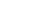subject to the condition that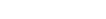.

### Prerequisites

Cauchy-Schwarz Inequality

Tangent-Normal

Conic section

## Solution :

This is a beautiful problem, but only if one notices the trick, or else things gets ugly.

Now we need to find the maximum ofwhen it is given that. Seeing the given condition we always think of using Lagrange Multipliers, but I find that thing very nasty, and always find ways to avoid it.

So let's recall the famous Cauchy-Schwarz Inequality,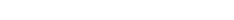.

Now, lets take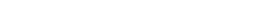, and observe our inequality reduces to,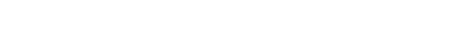. Hence the maximum ofwith respect to the given conditionis. Hence we got what we want without even doing any nasty calculations.

Another nice approach for doing this problem is looking through the pictures. Given the conditionrepresents a disc whose shape is elliptical, and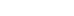is a family of straight parallel lines passing passing through that disc.

Hence the line with the maximum intercept among all the lines passing through the given disc represents the maximized value of. So, basically if a line of form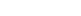(say), is a tangent to the disc, then it will basically represent the line with maximum intercept from the mentioned family of line. So, we just need to find the point on the boundary of the disc, where the line of formtouches as a tangent. Can you finish the rest and verify weather the maximum intercept .i.e.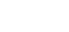or not.

## Food For Thought

Can you show another alternate solution to this problem ? No, Lagrange Multiplier Please !! How would you like to find out the point of tangency if the disc was circular ? Show us the solution we will post them in the comment.

Keep thinking !!

## Subscribe to Cheenta at Youtube

This is a very subtle sample problem from ISI MStat PSB 2006 Problem 2. After seeing this problem, one may think of using Lagrange Multipliers, but one can just find easier and beautiful way, if one is really keen to find one. Can you!

## Problem- ISI MStat PSB 2006 Problem 2

Maximizesubject to the condition that.

### Prerequisites

Cauchy-Schwarz Inequality

Tangent-Normal

Conic section

## Solution :

This is a beautiful problem, but only if one notices the trick, or else things gets ugly.

Now we need to find the maximum ofwhen it is given that. Seeing the given condition we always think of using Lagrange Multipliers, but I find that thing very nasty, and always find ways to avoid it.

So let's recall the famous Cauchy-Schwarz Inequality,.

Now, lets take, and observe our inequality reduces to,. Hence the maximum ofwith respect to the given conditionis. Hence we got what we want without even doing any nasty calculations.

Another nice approach for doing this problem is looking through the pictures. Given the conditionrepresents a disc whose shape is elliptical, andis a family of straight parallel lines passing passing through that disc.

Hence the line with the maximum intercept among all the lines passing through the given disc represents the maximized value of. So, basically if a line of form(say), is a tangent to the disc, then it will basically represent the line with maximum intercept from the mentioned family of line. So, we just need to find the point on the boundary of the disc, where the line of formtouches as a tangent. Can you finish the rest and verify weather the maximum intercept .i.e.or not.

## Food For Thought

Can you show another alternate solution to this problem ? No, Lagrange Multiplier Please !! How would you like to find out the point of tangency if the disc was circular ? Show us the solution we will post them in the comment.

Keep thinking !!

## Subscribe to Cheenta at Youtube

This site uses Akismet to reduce spam. Learn how your comment data is processed.

### One comment on “ISI MStat PSB 2006 Problem 2 | Cauchy & Schwarz come to rescue”

1.Spandan Ghoshal says:

The alternate solution can be using parametric representation of an Ellipse as it's evident that max(x+y) will be attained on the boundary points i.e on the ellipse itself. This reduces the bivariate function (x+y) into a single variate of \theta which makes the solution easy.

### Knowledge Partner